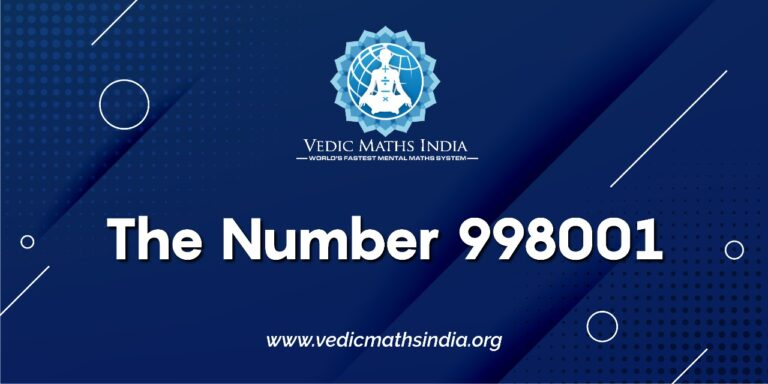# The Number 998001Recently, I showed some children in the library how to quickly multiply any three digit number by 999 using a very simple trick.

– First, subtract one from the number you wish to multiply by 999. Write down this number; it is the first half of the product.

So, for instance, if you were multiplying 568 by 999, the first thing you do is write down 567.

– Second, perform “All from 9 and the last from 10” on the number being multiplied by 999, and write this down next to the first three digits. That’s your number.

So, again if you were multiplying 568 by 999, performing Nikhilam on 568 yields 432. Putting that next to 567 yields 567432 – which is 568 x 999.

The number 998001 is actually 9992 = 999 x 999. (998001 is also the last square number before 1,000,000)

Again, working it out is easy once you know how to multiply three digit numbers by 999: subtract 1 from 999 to yield 998, and perform Nikhilam on 999 to yield 001.

998,001.

And now I can report on a delightful piece of information that I read about here:- namely, that the decimal fraction 1 / 998001 yields what the author calls “a surprising result” –According to the author, 1 / 998001 yields every 3 digit number from 000 through to 999, in order. Similarly, 1 / 9801 yields a decimal comprising every 2 digit number from 00 through 99 in order.

Incidentally, you can also any two digit figure by 99 using the exact same method as outlined above for multiplication by 999, only it yields a four digit number.

So, for instance, if you multiply 56 x 99, subtract 1 from 56 to yield 55, and perform Nikhilam on 56 to yield 44, which in turn yields the result 5544 for 56 x 99.

And what is 9801, but 99 x 99.

Two questions arise as a result of these revelations.

1. Does the number recur after 999 in the fraction 1 / 998001 (i.e. the fraction eventually reaches the sequence “… 990991992993994995996997998999” and repeats from “000”)? (The second half of the recurring portion would thus be a compliment of the first half, meaning that anyone computing 1 / 998001 would only need to calculate the first 1500 places after the decimal point) – and would the same result be true for 1 / 9081?

2. Is there a pattern here, which would hold true for 1 / 99980001 (9999 x 9999), 1 / 9999800001 (99999 x 99999), and so on? In other words, would we expect a recurring decimal part of 1 / 99980001 to begin “0.0000000100020003000400050006000700080009001000110012001300140015 …” and end with “… 9990999199929993999499959996999799989999” before repeating? How about “0.00000000010000200003000040000500006000070000800009 … 9999599996999979999899999” for the recurring part of the fraction 1 / 9999800001?